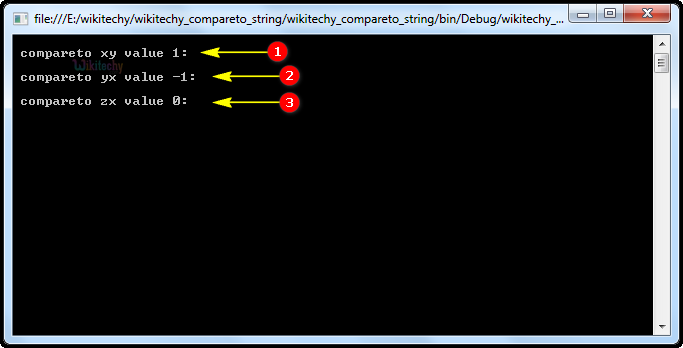# C# compareto | C# compareTo String | string compareto - c# - c# tutorial - c# netC# Compareto

## What is C# compareTo ?

• Compares this instance with a specified Object or string and returns an integer that indicates whether this instance precedes, follows, or appears in the same position in the sort order as the specified string or Object.
• Compare two strings and returns integer value as an output. It returns 0 for true and 1 for false.C# CompareTo String

## Syntax:

• Here o is representing the Object to be compared.
• anotherString is the String to be compared.

## Code Explanation:1. In this example we are using three integer variables x, y, z values as 10, 9, 10.
2. Here the variables "xy", "yx", and "zx" contain the results of the integer variables of "x", "y", and "z" compared to one another.
3. In Console.WriteLine, the Main method specifies its behavior with the string statement "compareto xy value" assigned in the xy variable values display on the screen. Similarly, yx, zx variables.

## Sample C# examples - Output :1. Here in this output, the (xy) first number x = 10 is larger than the y=9, so the result is 1 value is printed on the console.
2. In this output, the (yx) second number y = 9 is smaller than the x=10, so the result is -1 value is printed on the console.
3. In this output, the (zx) third number z=10 is equal to the x= 10, so the result is 0 values is printed on the console.